This tutorial makes use of the following R package(s): tidyr. Note that these functions can be used in a piping operation.

# Introduction

Data tables come in different sizes and shape; they can be a very simple two column dataset or they can consist of many columns and “sub-columns”. Understanding its structure, and learning how to reshape it into a workable form is critical to an effective and error free analysis.

For example, a median earnings data table downloaded from the U.S. census bureau’s website might look something like this: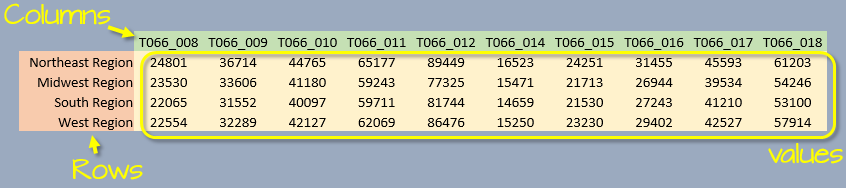We are conditioned to think of a table as consisting of three components: rows, columns and data values. Implicit in this paradigm is that each column represents a unique attribute. However, this may not always be the case. For example, in the above table, each column represents two distinct variables: gender and educational attainment (two distinct sets of attributes).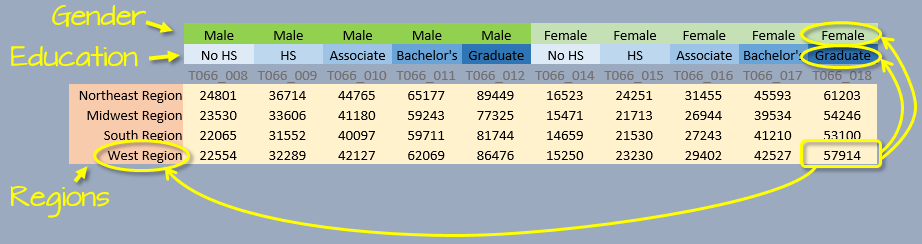Another way of describing a dataset is by defining its variable(s), values and observations. In the above example, we have four variables: gender, education, region and income. Each variable consists of either categorical values (e.g. region, gender and education) or numerical values (income).

An observation consists of a unique set of attribute values. For example the values West Region, Female, Graduate and \$57,914 make up one observation: there is just one instance of these combined values in the data. This perspective affords us another option in presenting the dataset: we can assign each column its own variable, and each row its own observation.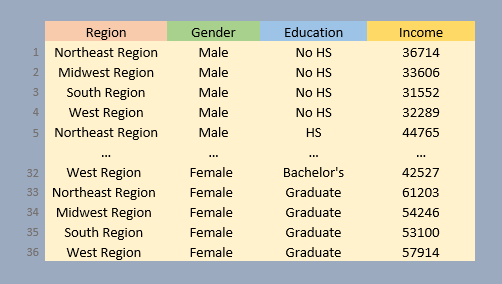Note that each row of the table is part of a unique set of variable attributes. A dataset in this format may not be human “readable” (unlike its wide counterpart), but is the format of choice for many data analysis and visualization operations.

The next sections will demonstrate how one can convert a wide format to a long format and vice versa.

# Wide and long table formats

A 2014 Boston (Logan airport) flight data summary table will be used in this exercise. The summary displays average mean delay time (in minutes) by day of the work week and quarter.

df <- data.frame( Weekday = c( "Mon", "Tues", "Wed", "Thurs", "Fri" ),
Q1      = c(  9.9 ,  4.9  ,  8.8 ,   12.2 ,  12.2 ),
Q2      = c(  5.4 ,  9.7  , 11.1 ,   10.2 ,   8.1 ),
Q3      = c(  8.8 ,  7.9  , 10.2 ,   9.2  ,   7.9 ),
Q4      = c(  6.9 ,    5  ,  9.3 ,   9.7  ,   5.6 ) )

Reshaping a table involves modifying its layout (or “shape”). In our example, df is in a “wide” format.

Weekday Q1 Q2 Q3 Q4
Mon 9.9 5.4 8.8 6.9
Tues 4.9 9.7 7.9 5.0
Wed 8.8 11.1 10.2 9.3
Thurs 12.2 10.2 9.2 9.7
Fri 12.2 8.1 7.9 5.6

There are three unique variables: day of week, quarter of year, and mean departure delay.

## Creating a long table from a wide table

A package that facilitates converting from wide to long (and vice versa) is tidyr. To go from wide to long, we use the gather function and from long to wide, we use the spread function.

The gather function takes three arguments:

• key: This is the name of the new column which will combine all column variables (e.g. Q1, Q2, Q3 and Q4).
• value: This is the name of the new column which will list all values (e.g. average delay times) associated with each variable combination (e.g. Mon and Q1, Tues and Q1, etc…)
• The third parameter does not have a name, but is a list of columns that are to be collapsed. The column can be referenced by column number or column name. Note that the column enumeration does not need be wrapped in a c() function.

In our example, the line of code needed to re-express the table into a long form can be written in one of three ways:

library(tidyr)
df.l <- gather(df, key = "Quarter", value = "Delay" , Q1, Q2, Q3, Q4 )
# or
df.l <- gather(df, key = "Quarter", value = "Delay" ,  2:5           )
# or
df.l <- gather(df, key = "Quarter", value = "Delay" ,  -Weekday      )

All three lines produce the same output, they differ only by how we are referencing the columns that are to be collapsed. Note that we assigned the names Quarter and Delay to the two new columns.

The first 10 lines of the output table are shown here. Note how each Delay value has its own row.

   Weekday Quarter Delay
1      Mon      Q1   9.9
2     Tues      Q1   4.9
3      Wed      Q1   8.8
4    Thurs      Q1  12.2
5      Fri      Q1  12.2
6      Mon      Q2   5.4
7     Tues      Q2   9.7
8      Wed      Q2  11.1
9    Thurs      Q2  10.2
10     Fri      Q2   8.1

The following figure summarizes the wide to long conversion.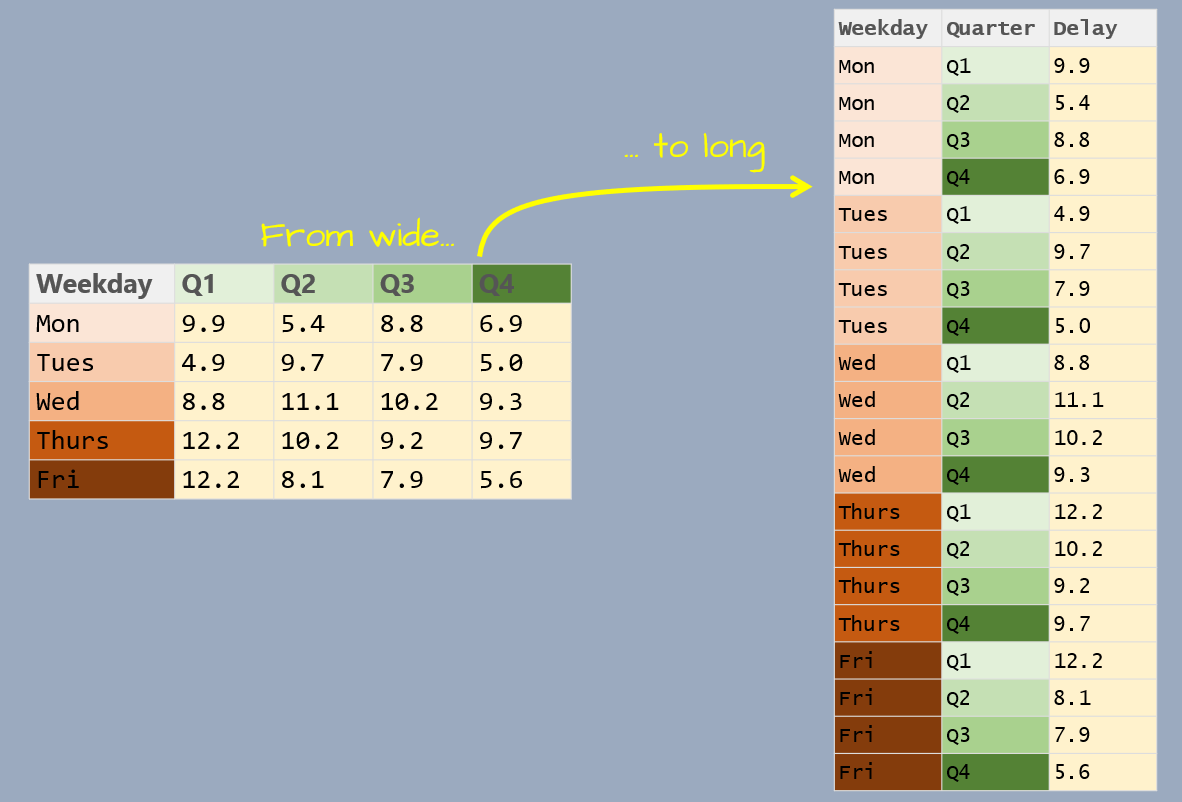## Creating a wide table from a long table

If a table is to be used for a visual assessment of the values, a long format may be difficult to work with. A long table can be re-expressed into a wide form by picking the two variables that will define the new unique rows and columns.

Continuing with our example, we will convert df.l back to a wide format.

df.w <-  spread( df.l ,   key = Quarter  ,   value = Delay  ) 

We’ve now recreated the wide version of our table.

  Weekday   Q1   Q2   Q3  Q4
1     Fri 12.2  8.1  7.9 5.6
2     Mon  9.9  5.4  8.8 6.9
3   Thurs 12.2 10.2  9.2 9.7
4    Tues  4.9  9.7  7.9 5.0
5     Wed  8.8 11.1 10.2 9.3

The following figure summarizes the long to wide conversion.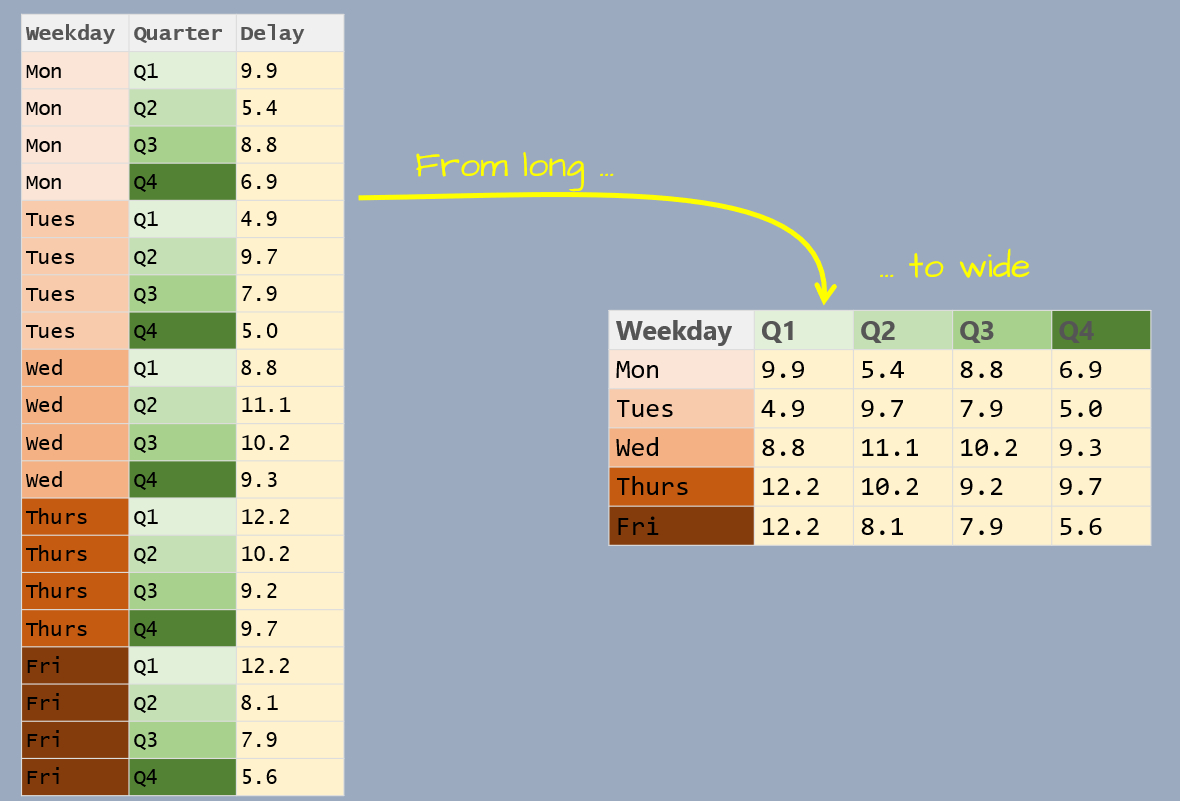# Combining elements from many columns into a single column

Another practical function in the tidyr package is unite(). It combines columns into a single column by chaining the contents of the combined columns. For example, the following table has hours, minutes and seconds in separate columns.

library(tidyr)
df <- data.frame(
Index = c( 1,2,3),
Hour = c(2,14,20),
Min = c(34,2,55),
Sec = c(55, 17, 23))

df
  Index Hour Min Sec
1     1    2  34  55
2     2   14   2  17
3     3   20  55  23

To combine the three time elements into a single column, type:

df2 <- unite(df,  col = Time , 2:4, sep=":", remove=TRUE)
df2
  Index     Time
1     1  2:34:55
2     2  14:2:17
3     3 20:55:23

The col parameter defines the new column name; the paremeter 2:4 tells unite that columns two through four are to be combined into column Time; sep=":" tells the function what characters are to be used to separate the elements (here, we are separating the time elements using :); remove=TRUE tells the function to remove columns two through four.

# Separating elements in one column into separate columns

The reverse of unite() is separate(). Continuing with the last example, if we want to split the time elements into their respective columns, type:

df3 <-  separate(df2,  col = Time , c("Hour", "Minute", "Second"), sep=":", remove=TRUE)
df3
  Index Hour Minute Second
1     1    2     34     55
2     2   14      2     17
3     3   20     55     23

You will recognize many of the parameters from the unite function with one difference: the new (to be created) column names need to be defined in a combine, c(), statement.

# Filling gaps in a table using complete

It’s not uncommon to be handed a table with incomplete combinations of observations. For example, the following table gives us yield and data source values for each combination of year and grain type. However, several combinations of year/grain are missing.

df <- data.frame( Year  = c(1999,1999,2000,2000,2001,2003,2003,2005),
Grain = c("Oats", "Corn","Oats", "Corn","Oats", "Oats", "Corn","Oats"),
Yield = c(23,45,24,40,20,19,41,22),
Src   = c("a","a","b","c","a","a","c","a"))
df
  Year Grain Yield Src
1 1999  Oats    23   a
2 1999  Corn    45   a
3 2000  Oats    24   b
4 2000  Corn    40   c
5 2001  Oats    20   a
6 2003  Oats    19   a
7 2003  Corn    41   c
8 2005  Oats    22   a

For example, we do not have records for 2001 and Corn, 2003 and Corn, and data for both grains are missing for 2002 and 2004. To add rows for all missing pairs of year/grain values, use the complete function.

df.all <- complete(df, Year=1999:2005, Grain=c("Oats", "Corn"), fill=list(Yield=NA,Src=NA))
df.all
# A tibble: 14 × 4
Year Grain Yield    Src
<dbl> <chr> <dbl> <fctr>
1   1999  Corn    45      a
2   1999  Oats    23      a
3   2000  Corn    40      c
4   2000  Oats    24      b
5   2001  Corn    NA     NA
6   2001  Oats    20      a
7   2002  Corn    NA     NA
8   2002  Oats    NA     NA
9   2003  Corn    41      c
10  2003  Oats    19      a
11  2004  Corn    NA     NA
12  2004  Oats    NA     NA
13  2005  Corn    NA     NA
14  2005  Oats    22      a

The function allows you to define the full range of expected values for year and Grain; the function will then create all pair combinations and add those pairs that are missing to the table. For all missing pairs, the function allows the user to define the missing values to assign to the Yield and Src variables (here, we choose to assign NA values to each).

Note that the combine function can be used with one or more columns in a table.